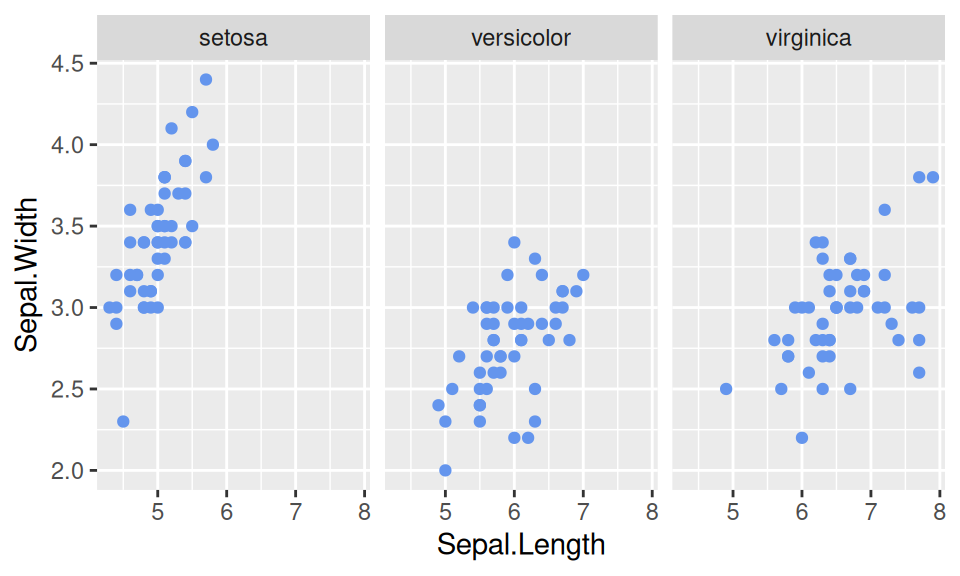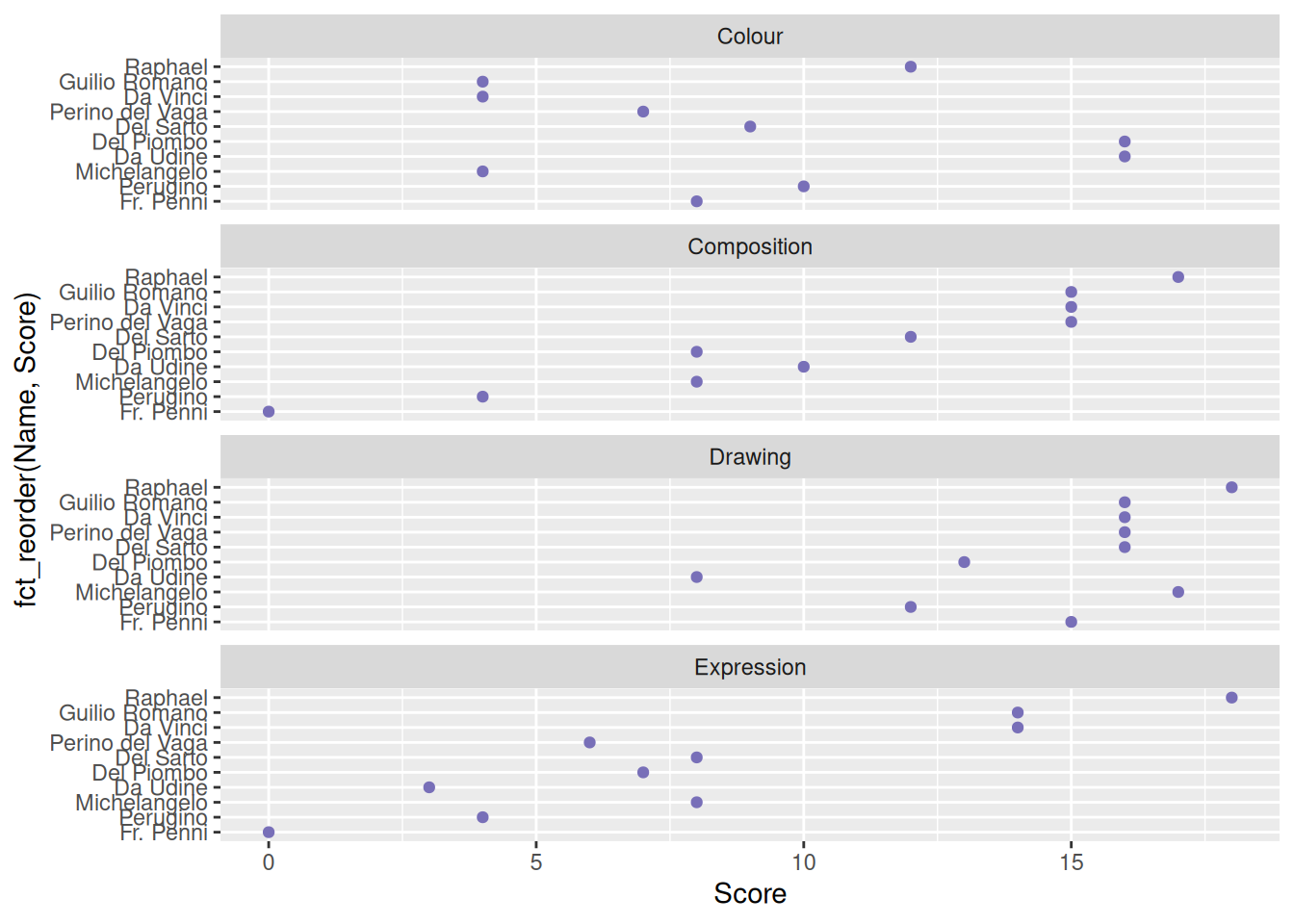# Chapter 8 Faceting

In this chapter, we will introduce facets, which are usually used to combine continuous and categorical data.

## 8.1 Faceting on one variable

Facet partitions a plot into a matrix of panels. Each panel shows a different subset of the data. By default, `facet_wrap` gives consistent scales, which is easier for comparison between different panels.

``````library(ggplot2)
mycol = "#7192E3"
ggplot(iris, aes(Sepal.Length, Sepal.Width)) +
geom_point(color = mycol) +
facet_wrap(~Species) +
theme_grey(18)``````Rather than faceting on factor level, we can have one panel for each numerical variable.

``````library(pgmm)
library(dplyr)
library(tidyr)
data(wine)
tidywine <- wine %>%
pivot_longer(cols = -Type, names_to = "variable", values_to = "value")
tidywine %>%
ggplot(aes(value)) +
geom_histogram() +
facet_wrap(~variable) +
ggtitle("Consistent scales") +
theme_grey(14)``````Axis scales can be made independent, by setting scales to `free`, `free_x`, or `free_y`.

In this case, `scales = "free_x"` is a better option because the distribution of each numerical variable is more obvious.

``````tidywine  %>%
ggplot(aes(value)) +
geom_histogram() +
facet_wrap(~variable,scales = "free_x") +
ggtitle("Consistent scales") +
theme_grey(14)``````## 8.2 Faceting on two variables

`facet_grid` can be used to split data-sets on two variables and plot them on the horizontal and/or vertical direction.

``````wine %>%
mutate(Type = paste("Type", Type)) %>%
select(1:6) %>%
pivot_longer(cols = -Type, names_to = "variable", values_to = "value") %>%
ggplot(aes(value)) +
geom_histogram(color = mycol, fill = "lightblue") +
facet_grid(Type ~ variable, scales = "free_x") +
theme_grey(14)``````